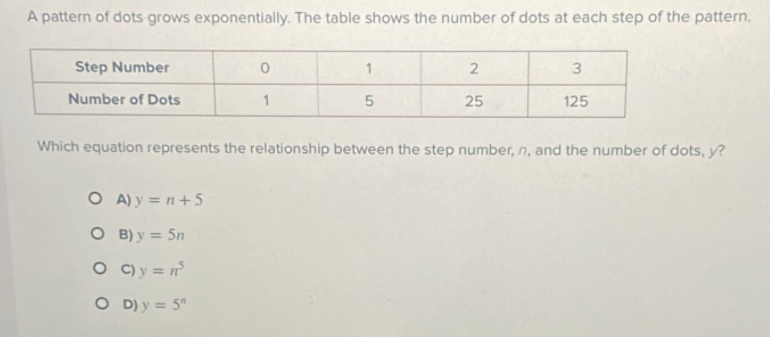### ¿Todavía tienes preguntas de matemáticas?

Pregunte a nuestros tutores expertos
Algebra
PreguntaA pattern of dots grows exponentially. The table shows the number of dots at each step of the pattern.  Which equation represents the relationship between the step number, $$n ,$$ and the number of dots, $$y ?$$

A) $$y = n + 5$$

B) $$y = 5 n$$

C) $$y = n ^ { 5 }$$

D) $$y = 5 ^ { n }$$